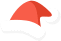WordPress как на ладонифункция не описана

# WC_Cart_Totals::calculate_discounts() protectedWC 3.2.0

Calculate COUPON based discounts which change item prices.

{} Это метод класса: WC_Cart_Totals{}

Хуков нет.

Null. Ничего.

### Использование

```// protected - в коде основоного (родительского) или дочернего класса
\$result = \$this->calculate_discounts();```

### Список изменений

 С версии 3.2.0 Введена.

### Код WC_Cart_Totals::calculate_discounts() WC Cart Totals::calculate discounts WC 4.7.1

```protected function calculate_discounts() {
\$this->get_coupons_from_cart();

\$discounts = new WC_Discounts( \$this->cart );

// Set items directly so the discounts class can see any tax adjustments made thus far using subtotals.
\$discounts->set_items( \$this->items );

foreach ( \$this->coupons as \$coupon ) {
\$discounts->apply_coupon( \$coupon );
}

\$coupon_discount_amounts     = \$discounts->get_discounts_by_coupon( true );
\$coupon_discount_tax_amounts = array();

// See how much tax was 'discounted' per item and per coupon.
if ( \$this->calculate_tax ) {
foreach ( \$discounts->get_discounts( true ) as \$coupon_code => \$coupon_discounts ) {
\$coupon_discount_tax_amounts[ \$coupon_code ] = 0;

foreach ( \$coupon_discounts as \$item_key => \$coupon_discount ) {
\$item = \$this->items[ \$item_key ];

if ( \$item->product->is_taxable() ) {
// Item subtotals were sent, so set 3rd param.
\$item_tax = wc_round_tax_total( array_sum( WC_Tax::calc_tax( \$coupon_discount, \$item->tax_rates, \$item->price_includes_tax ) ), 0 );

// Sum total tax.
\$coupon_discount_tax_amounts[ \$coupon_code ] += \$item_tax;

// Remove tax from discount total.
if ( \$item->price_includes_tax ) {
\$coupon_discount_amounts[ \$coupon_code ] -= \$item_tax;
}
}
}
}
}

\$this->coupon_discount_totals     = (array) \$discounts->get_discounts_by_item( true );
\$this->coupon_discount_tax_totals = \$coupon_discount_tax_amounts;

if ( wc_prices_include_tax() ) {
\$this->set_total( 'discounts_total', array_sum( \$this->coupon_discount_totals ) - array_sum( \$this->coupon_discount_tax_totals ) );
\$this->set_total( 'discounts_tax_total', array_sum( \$this->coupon_discount_tax_totals ) );
} else {
\$this->set_total( 'discounts_total', array_sum( \$this->coupon_discount_totals ) );
\$this->set_total( 'discounts_tax_total', array_sum( \$this->coupon_discount_tax_totals ) );
}

\$this->cart->set_coupon_discount_totals( wc_remove_number_precision_deep( \$coupon_discount_amounts ) );
\$this->cart->set_coupon_discount_tax_totals( wc_remove_number_precision_deep( \$coupon_discount_tax_amounts ) );

// Add totals to cart object. Note: Discount total for cart is excl tax.
\$this->cart->set_discount_total( \$this->get_total( 'discounts_total' ) );
\$this->cart->set_discount_tax( \$this->get_total( 'discounts_tax_total' ) );
}```